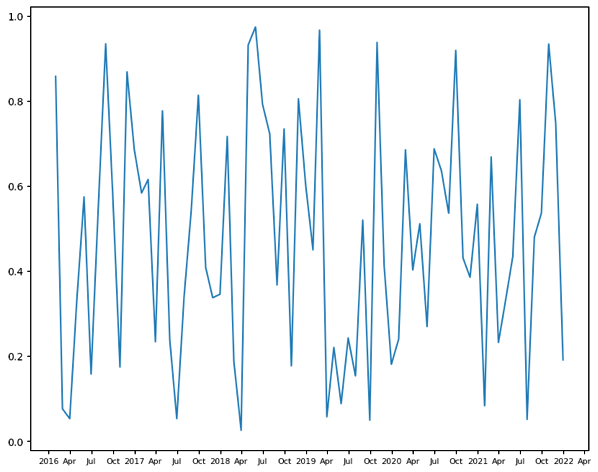# Format time axis to "2020-JAN-2021-JAN"

I’m trying to find a way to format the datetime axis to display years as numbers and months as uppercase letters. Unfortunately, Python’s `strptime` function doesn’t provide uppercase month names (e.g. APR). I need to format it myself, but I haven’t been able to figure out how. Since I only want to show Apr, Jul, Oct. I used a `MonthLocator` in a `ConciseDateFormatter`. I have tried the following:

``````import pandas as pd
import numpy as np

import matplotlib.pyplot as plt
from matplotlib.dates import MonthLocator
from matplotlib.dates import ConciseDateFormatter
from matplotlib.ticker import FuncFormatter

x = pd.date_range('2016-01-01', '2021-12-31', freq='M')
y = np.random.rand(len(x))

fig, ax = plt.subplots(figsize=(10, 8))
locator = MonthLocator((1, 4, 7, 10))
ax.xaxis.set_major_locator(locator)
ax.xaxis.set_major_formatter(
ConciseDateFormatter(
locator, formats=['%Y', '%b', '%d', '%H:%M', '%H:%M', '%S.%f']
)
)
ax.tick_params(axis='x', labelsize=8)
ax.plot(x, y)
``````But I want the axis to be

``````2016 - APR - JUL - OCT - 2017 - APR - JUL -OCT - 2018 -
``````

I’ve tried a few ways, but none of them works. Once I use a user-defined `FuncFormatter`, it will no longer respect my `ConciseDateFormatter`. I’m wondering what the easiest way is for me to achieve my desired outcome. Should I simply write a formatter function that includes an if statement? If the year and month are both equal to 1, it should display the year number; otherwise, it should show the month name. If this is the case, how can I accomplish it?

Have you tried using set_ticklabels? Something like

``````ax.tick_params(axis='x', labelsize=8)
ax.plot(x, y)
ax.xaxis.set_ticklabels([t.get_text().upper()
for t in ax.get_xticklabels()
]);

``````

Matplotlib issues a UserWarning: set_ticklabels() should only be used with a fixed number of ticks, i.e. after set_ticks() or using a FixedLocator, so this may not work in all circumstances.

Thank you! Indeed, I believe this is a way to format the labels instead of resetting them manually.

1 Like

Another approach would be to create a ConciseDateFormatter subclass that overrides format_ticks. This approach may not work for a wide range of Matplotlib versions and could break if the ConciseDateFormatter code changes in a future update. This works in Matplotlib 3.7.3 and 3.8.0

``````import pandas as pd
...
from matplotlib.dates import ConciseDateFormatter
import calendar   # for month names in your locale

class UpperConciseDateFormatter(ConciseDateFormatter):
def format_ticks(self, values):
labels = super().format_ticks(values)
if not self._usetex:
labels =  [label.upper()
for label in labels]
else:
months = [calendar.month_abbr[m] for m in range(1, 13)]
labels = [label.upper() if label in months else label
for label in labels]
return labels

x = pd.date_range('2016-01-01', '2021-12-31', freq='M')
...
ax.xaxis.set_major_formatter(
UpperConciseDateFormatter(
locator, formats=['%Y', '%b', '%d', '%H:%M', '%H:%M', '%S.%f']
)
)
ax.tick_params(axis='x', labelsize=8)
ax.plot(x, y)

``````
1 Like